• 动态编程在计算机编程中是一种技术，能够有效解决拥有重复子问题的一类问题，这类问题通常需要重复计算子问题的值。 例子 以斐波那契数列问题为例说明动态编程 如果序列是F(1) F(2) F(3)…F(50)，遵循F(n) = F(n-1) ...
介绍
动态编程在计算机编程中是一种技术，能够有效解决拥有重复子问题的一类问题或者最佳子结构的问题。
例子
以斐波那契数列问题为例说明动态编程
如果序列是F(1) F(2) F(3)…F(50)，遵循F(n) = F(n-1) + F(n-2)
F(50) = F(49) + F(48)
F(49) = F(48) + F(47)
F(48) = F(47) + F(46)
...

注意到拥有重复子问题，我们需要计算F(48)来计算F(50)和F(49)。动态编程可以解决的类似这样的问题。
工作原理
动态编程通过存储子问题的结果，以至当子问题的结果需要时，可以直接使用，而不需要重新计算。
存储子问题的值到数组中，节省重新计算的时间。
var m = map(0 → 0, 1 → 1)
function fib(n)
if key n is not in map m
m[n] = fib(n − 1) + fib(n − 2)
return m[n]

上面的动态编程是一种自上而下的动态编程。但是通过反转算法，从基数开始计算，使用自下而上的动态编程
function fib(n)
if n = 0
return 0
else
var prevFib = 0, currFib = 1
repeat n − 1 times
var newFib = prevFib + currFib
prevFib = currFib
currFib  = newFib
return currentFib

递归 vs 动态编程
动态编程大部分可以应用到递归算法，大部分优化问题需要递归和动态编程一起使用进行优化。
但并不是所有使用递归的问题可以使用动态编程，除非有重复子问题的存在，像斐波那契数列问题。像合并排序算法是不能使用动态编程的递归方法，因为没有重复的子问题。递归实际上使用分而治之的方法来解决问题。
贪婪算法 vs 动态编程
贪婪算法和动态编程都是优化问题的工具。
但是贪婪算法主要寻找局部优化，但是不能保证全局优化。
动态编程寻找最佳的方法解决子问题，然后利用这些子问题的结果来找到最佳的解决方法。


展开全文• 动态编程语言静态编程语言 介绍 (Introduction) Today in this tutorial, we are going to discuss the concept of Dynamic Programming. It is a much more optimal approach to programming. 今天，在本教程中，...
动态编程语言静态编程语言 介绍 (Introduction)
Today in this tutorial, we are going to discuss the concept of Dynamic Programming. It is a much more optimal approach to programming. 今天，在本教程中，我们将讨论动态编程的概念。 这是一种更好的编程方法。

动态编程 (Dynamic Programming)
Theoretically, Dynamic Programming is a problem-solving technique that solves a problem by dividing it into sub-problems. When the sub-problems are same and dependent, Dynamic programming comes into the picture. 从理论上讲， 动态编程是一种解决问题的技术，它通过将问题分成子问题来解决。 当子问题相同且相互依赖时，动态编程就会出现。

It is very similar to the Divide and Conquer approach to problem-solving. But in some cases, the Divide and Conquer technique may perform some operations or tasks multiple times (increasing time complexity). 它与解决问题的“ 分而治之”方法非常相似。 但是在某些情况下，分而治之技术可能会多次执行某些操作或任务（增加时间复杂度）。
Hence, the Dynamic Programming method (DP) is normally used to optimize a specific problem. 因此，动态编程方法（DP）通常用于优化特定问题。
Further, DP follows the Principle of Optimality which states that for an optimal sequence of decisions, each sub-sequence must also be optimal. 此外， DP遵循最优性原则，该原则指出，对于最佳决策序列，每个子序列也必须是最优的。

示例–使用DP的斐波那契 (Example – Fibonacci Using DP )
Now that we have discussed the basic concept of Dynamic Programming, let us look at how to solve a problem using this method. 现在我们已经讨论了动态编程的基本概念，让我们看看如何使用这种方法解决问题。
In the example below, we try to calculate the number at the position n (user input) in the Fibonacci series. 在下面的示例中，我们尝试计算斐波那契数列中位置n （用户输入）处的数字。
As you know, a Fibonacci series looks like this, 如您所知，斐波那契数列看起来像这样，
0, 1, 1, 2, 3, 5, 8 … upto n terms. 0、1、1、2、3、5、8…最多n个词。
Here, the starting two digits are 0 and 1. 在这里，起始两位数字是0和1 。
Let’s write a program to automatically find the right number at a specific point in the Fibonacci sequence based on the user input. 让我们编写一个程序，根据用户输入在斐波那契数列的特定点自动找到正确的数字。

Using Recursion(in C): 使用递归（在C中）：

#include<stdio.h>
//fibonacci calculating function
int fibo(int n)
{
if(n==1)
return 0;
else if(n==2)
return 1;
else
return fibo(n-1) + fibo(n-2);
}
int main()
{
int n,res;
printf("Enter n : ");
scanf("%d",&n);

res=fibo(n);

printf("fibo(%d) = %d",n,res);

return 0;
}
Output: 输出 ：

Enter n : 5
fibo(5) = 3Fibonacci using Recursion( if n=5 )So as you can see, in our above example taking n=5, recursion breaks the problem into sub-problems using recursive calls. 如您所见，在我们上面的示例中，使用n=5 ，递归使用递归调用将问题分解为子问题。
Take a closer look, notice that in this case, fibo(3) is calculated twice. Which then breaks down to two recursive calls for fibo(1) and fibo(2). They have values 0 and 1 respectively. Finally, we get our desired output i.e., fibo(5) = 3. 仔细观察一下，请注意，在这种情况下， fibo(3)是两次计算的。 然后将其分解为对fibo(1)和fibo(2)两个递归调用。 它们的值分别为0和1 。 最后，我们得到所需的输出，即fibo(5) = 3 。

Applying DP(in C): 应用DP（在C中）：

#include<stdio.h>

int fibo_series;

void set_zero()
{
int i;
for (i = 1; i <= 100; i++)
fibo_series[i] = 0;
}
//fibonacci calculating function
int fibo(int n)
{
if (fibo_series[n] == 0)
{
if (n <= 1)
fibo_series[n] = n;
else
fibo_series[n] = fibo(n - 1) + fibo(n - 2);
}
return fibo_series[n];
}
int main ()
{
int n,res;
set_zero();

printf("Enter n : ");
scanf("%d",&n);

if(n>=100 || n<=0)
printf("Entered number is out of range!");
else
{
res=fibo(n-1);
printf("fibo(%d) = %d",n,res);
}

return 0;
}
Output: 输出 ：

Enter n : 5
fibo(5) = 3
This time firstly we initialize an array fibo_series[] with a maximum 100 elements to have a value 0, using the function set_zero(), 这次我们首先使用函数set_zero()初始化一个数组fibo_series[] ，该数组最多包含100个元素，值为0 。 This array is further going to be used to store the Fibonacci series’s elements as soon as one is calculated, 一旦计算出该数组，该数组将进一步用于存储斐波那契数列的元素， Inside the fibo() function first, we check whether the required element has been already calculated and stored inside the array or not. If not, it is calculated. And if yes, the element is directly passed to the site of the function call, 首先在fibo()函数内部，我们检查所需的元素是否已经计算并存储在数组中。 如果不是 ，则进行计算。 如果是 ，则将元素直接传递到函数调用的位置， This ensures that the same function(as we saw earlier fibo(3)) is not called twice. Hence in this way, we get the optimal solution. 这样可以确保同一函数（如我们先前看到的fibo（3）） 不会被调用两次。 因此，通过这种方式，我们获得了最佳解决方案。 Note: We pass (n-1) while calculating res to the function fibo() since the array index starts from zero. Whereas, we are considering the elements of the series to start with index 1. 注意：由于数组索引从零开始，因此在计算res时将(n-1)传递给函数fibo() 。 鉴于我们正在考虑从索引1开始的系列元素。
For the same reason, we have used an if-else statement to eliminate the cases where the user enters n as n>=100 or n<=0. 出于相同的原因，我们使用了if-else语句来消除用​​户以n>=100或n<=0输入n的情况。

结论 (Conclusion)
So in this tutorial, we learned about the Dynamic Programming technique with its implementation and how it gives us an optimal solution. 因此，在本教程中，我们学习了动态编程技术及其实现方法，以及它如何为我们提供最佳解决方案。
For any further questions, feel free to use the comments below. 如有其他疑问，请随时使用以下评论。

参考资料 ()
展开全文• 动态编程
【上一篇 3 有限马尔可夫决策过程（Finite Markov Decision Processes）】
【下一篇 5 蒙特卡洛方法 （Monte Carlo Method） 】
之前介绍的知识都是基础，从这次开始才真正开始介绍增强学习的解法方法。
动态编程（Dynamic Programming, DP）这个词大家肯定都不陌生，在解决算法编程问题当中经常会用到，它的主要思想就是将一个复杂的问题分解成多个子问题，将子问题的解结合在一起就构成了原问题的解，它常常适合于解决具有如下两种属性的问题：
（1）优化的子结构：优化解常常可以分解成子问题；
（2）子问题有重叠：即子问题总是重复出现，该子问题的解可以保存下来重复利用。

而马尔可夫决策过程就完美的符合这两点特性：
（1）需要求解的 Bellman 方程提供了递归的分解形式；
（2）Value function 需要存储和重用之前的解。
因此可以说 DP 可以用于求解马尔可夫模型的优化策略，但是由于 DP 问题需要环境的动态模型，并且计算复杂度较高，因此在增强学习中的应用并不广泛，但是它的思想在理论上还是非常重要的，是以后学习的一个理论基础。DP 算法的主要思想就是利用 value function 来寻找好的策略。

这里先介绍一下后文中的符号表示（与之前的相同）：
假设状态集合、行为集合和 reward 集合分别为 $\mathcal{S}$、$\mathcal{A}(s)$ 和 $\mathcal{R}$，动态性用概率 $p(s',r|s,a)$ 来表示，其中 $s\in\mathcal{S}$、$a\in\mathcal{A}(s)$、$r\in\mathcal{R}$、$s'\in\mathcal{S}^+$（若问题为 episodic，则 $\mathcal{S}^+$ 指的是 $\mathcal{S}$ 加上终止状态）。
之前介绍过，若找到了满足 Bellman 优化方程的优化值函数 $v_*$ 或者 $q_*$，就可以容易的获得优化的策略，$v_*$ 与 $q_*$ 的表达式如下所示：
\begin{aligned} v_{\ast}(s) &= \max_a{\Bbb E}[R_{t+1}+\gamma v_{\ast}(S_{t+1})|S_t=s,A_t=a] \\ &= \max_a\sum_{s’,r}{p(s',r|s,a)[r+\gamma v_{\ast}(s')]} \end{aligned}
\begin{aligned} q_{\ast}(s,a) &= {\Bbb E}[R_{t+1}+\gamma \max_{a'}q_{\ast}(S_{t+1},a') |S_t=s, A_t=a] \\ &= \sum_{s’,r}{p(s',r|s,a)[r+\gamma \max_{a'}q_{\ast}(s',a')]} \end{aligned}
1 Policy Evaluation
在DP中，Policy Evaluation 指的是对任意规则 $\pi$ 计算 state-value 函数 $v_{\pi}$，也可以将这个称作是 预测问题（Prediction Problem）。在上一节的内容中提到 $s\in\mathcal{S}$ 都有
\begin{aligned} v_{\pi}(s) &\doteq {\Bbb E}_{\pi}[R_{t+1}+\gamma R_{t+2}+\gamma ^2 R_{t+3}+\cdots | S_t=s] \\ &= {\Bbb E}_{\pi}[R_{t+1}+\gamma v_{\pi}(S_{t+1})| S_t=s] \\ &=\sum_a{\pi(a|s)}\sum_{s',r}{p(s',r|s,a)[r+\gamma v_{\pi}(s')]} \end{aligned}
从最后一个式子中可以看出，$v_{\pi}(s)$ 的计算还要依赖于 $v_{\pi}(s')$，对于这种情况，最常用的方法采用迭代的形式，不断地更新和逼近真实值。这里可以考虑一个近似的值函数序列 $v_0,v_1,v_2,\cdots$，其中每个值函数都是从 $\mathcal{S}^+$ 向 ${\Bbb R}$ 的映射，其中初始的近似值 $v_0$ 是任意选择的（终止状态的值为 0），之后的计算都是利用 $v_{\pi}$ 的 Bellman 方程来进行更新的：
\begin{aligned} v_{k+1}(s) &\doteq {\Bbb E}_{\pi}[R_{t+1}+\gamma v_{k}(S_{t+1})| S_t=s] \\ &=\sum_a{\pi(a|s)}\sum_{s',r}{p(s',r|s,a)[r+\gamma v_{k}(s')]} \end{aligned}
当 $k \rightarrow \infty$ 时，序列 $\{v_k\}$ 会收敛于 $v_{\pi}$，这个算法就称为是 Iterative Policy Evaluation。整个过程可以描述为:
$v_0 \rightarrow v_1 \rightarrow v_2 \rightarrow \cdots \rightarrow v_{\pi}$
为了从 $v_k$ 得到近似的 $v_{k+1}$，iterative policy evaluation 需要对每一个状态 $s$ 做同样的操作：将 $s$ 旧的 value 值换成计算得到的新的 value 值，将这种操作可以称为是 Full Backup，迭代策略评估的每一次迭代过程都需要把每个状态的 value 备份起来。
迭代算法常用的停止准则是检测最大的状态变化量，整个过程的伪代码如下：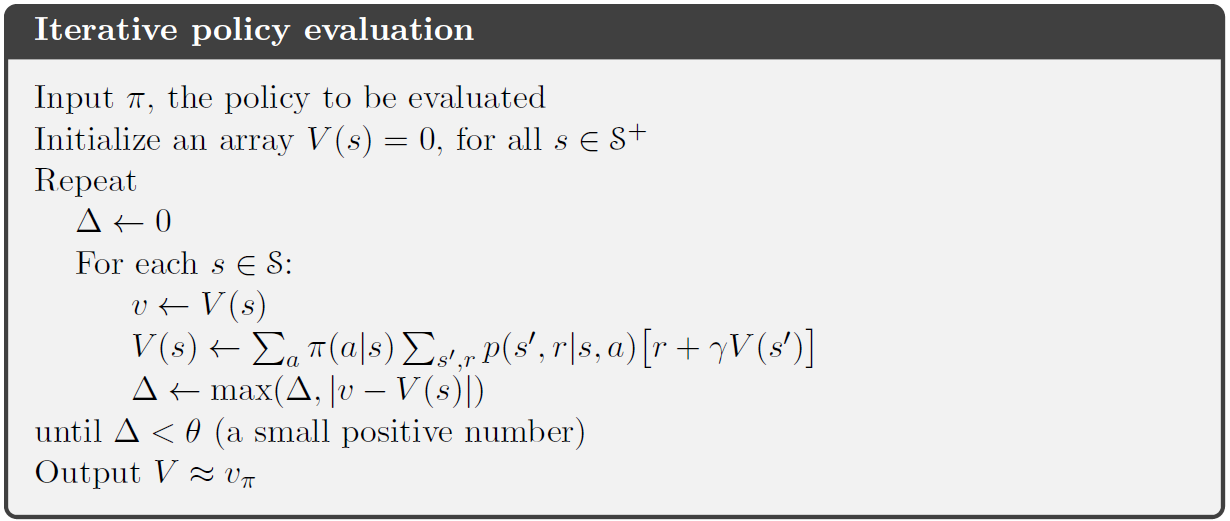2 Policy Improvement
第一节中是计算每个 policy 的 value function，它的目的当然是为了找到更好的 policies，假设我们已经有了某个 $\pi$ 的值函数 $v_{\pi}$，那我们肯定想知道是否可以通过对规则 $\pi$ 改进来得到更好的规则，改进的方式就是在改变在某个状态时的行为选择概率，即 $a=\pi(s)$，我们肯定会想，在状态 $s$ 时，我们依据当前的 $v_{\pi}(s)$ 到底是好还是不好呢？是否可以改进呢？那我们可以采用比较 $q_{\pi}(s,a)$ 与 $v_{\pi}(s)$ 的方法，若 $q_{\pi}(s,a)$ 比 $v_{\pi}(s)$ 大，那么采用 “在状态 $s$ 时选择行为 $a$，之后还遵从规则 $\pi$ ” 就要比 “无论什么状态下都遵从规则 $\pi$” 要好。

通常将这种理论称为是 Policy Improvement Theorem，假设一对任意的规则 $\pi$ 与 $\pi'$，若对所有的 $s\in\mathcal{S}$ 都有：
$q_{\pi}(s,\pi'(s)) \geq v_{\pi}(s)$
那么规则 $\pi'$ 一定比规则 $\pi$ 更好或者相当，也就是说，对所有的状态 $s\in\mathcal{S}$ 它的期望返回值都要高或者相当：
$v_{\pi'}(s) \geq v_{\pi}(s)$
回到我们之前的问题，我们如何对规则 $\pi$ 进行改进呢？这里假设规则 $\pi$ 是原始的规则，规则 $\pi '$ 是改进后的规则，这两个规则只有在 $\pi'(s)=a \neq \pi(s)$ 不同，其他地方完全相同，若我们知道 $q_{\pi}(s,a)> v_{\pi}(s)$，那我们就可以知道规则 $\pi$ 比规则 $\pi$ 要好，它的证明过程如下：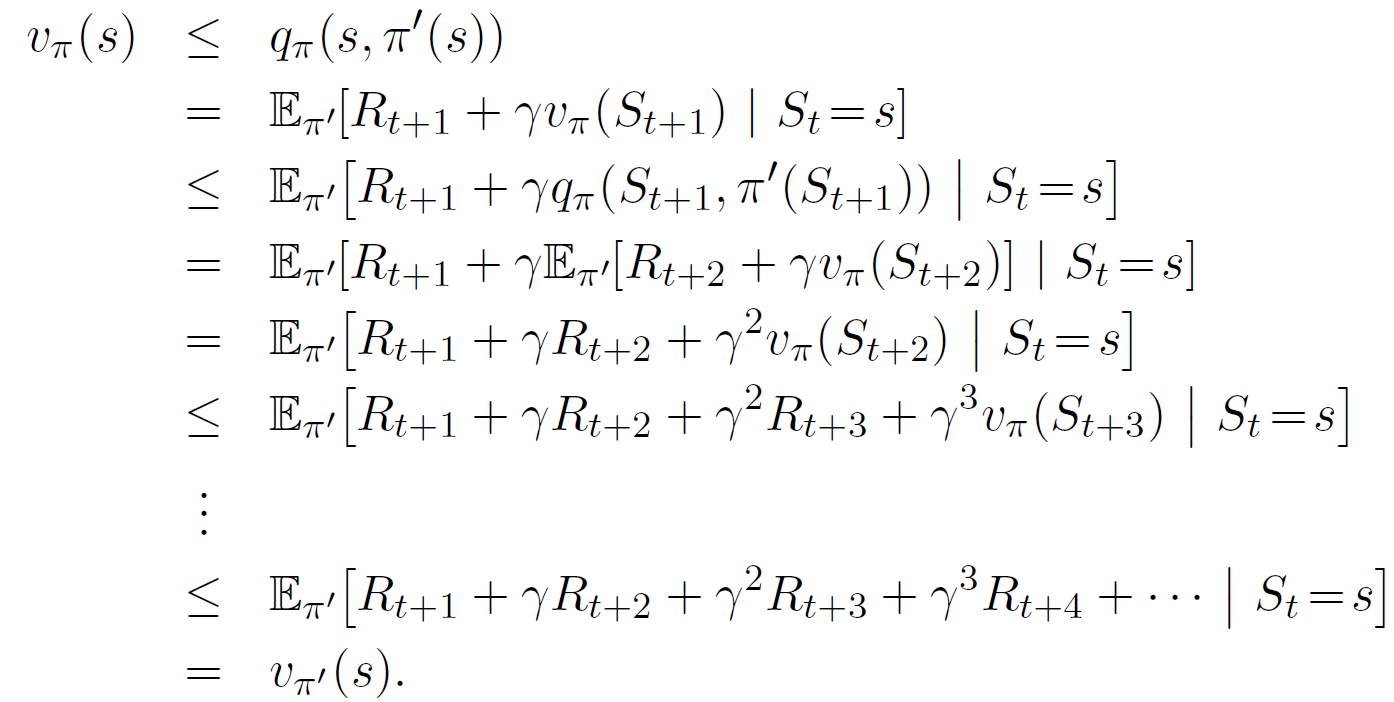从中可以看出，只要改善一个状态的行为就可以对规则进行改进，自然而然地肯定会想，如果把所有的状态都改成可能的最好行为，那得到的规则会很好，也就是这样的一个 greedy 规则 $\pi$：
\begin{aligned} {\pi}'(s) &\doteq arg\max_a{q_{\pi}(s,a)} \\ &= arg\max_a {\Bbb E} [R_{t+1}+\gamma v_{\pi}(S_{t+1})| S_t=s,A_t=a] \ \ \ \ \ \ \ (1)\\ &= arg\max_a \sum_{s',r}p(s',r|s,a)[r+\gamma v_{\pi}(s')] \end{aligned}
其中 $arg\max_a$ 指的是选择使后面式子最大的行为 $a$。
这种贪婪的规则依据 $v_{\pi}$ 选择短期内最好的行为，从结构上看，这种贪婪的规则满足 policy improvement theorem，因此比原始的规则要好，因此将这个过程就称为是 Policy Improvement。
假设这个贪婪的规则 $\pi'$ 并不比规则 $\pi$ 好，而是两者相当，则$v_{\pi}= v_{\pi'}$，则根据公式（1）有：
\begin{aligned} v_{\pi'}(s) &= \max_a {\Bbb E} [R_{t+1}+\gamma v_{\pi'}(S_{t+1})| S_t=s,A_t=a] \\ &= \max_a \sum_{s',r}p(s',r|s,a)[r+\gamma v_{\pi'}(s’)] \end{aligned}
这个方程与 Bellman 优化方程相同，因此 $v_{\pi'}(s)$ 一定是 $v_{\ast}$，因此规则 $\pi'$ 与规则 $\pi$ 一定都是 optimal policies。因此，除非该规则已经是 optimal policies，否则 policy improvement 过程一定会给出一个严格更好的规则。
3 Policy Iteration
第一节介绍的是规则评价，第二节是规则改善，这里要将前两节结合到一起，从而得到下面的过程：
$\pi_0 \xrightarrow{E} v_{\pi_0} \xrightarrow{I} \pi_1 \xrightarrow{E} v_{\pi_1} \xrightarrow{I} \pi_2 \xrightarrow{E} \cdots \xrightarrow{I} \pi_{\ast} \xrightarrow{E} v_{\pi_{\ast}}$
其中，$\xrightarrow{E}$ 代表的是 policy evaluation，$\xrightarrow{I}$ 代表的是 policy improvement，并且该 improvement 保证是严格的提升。因为有限 MDP 只含有有限的规则，因此该过程最终一定会收敛到 optimal policy。这种查询过程就称为是 Policy Iteration，完整的伪代码如下所示：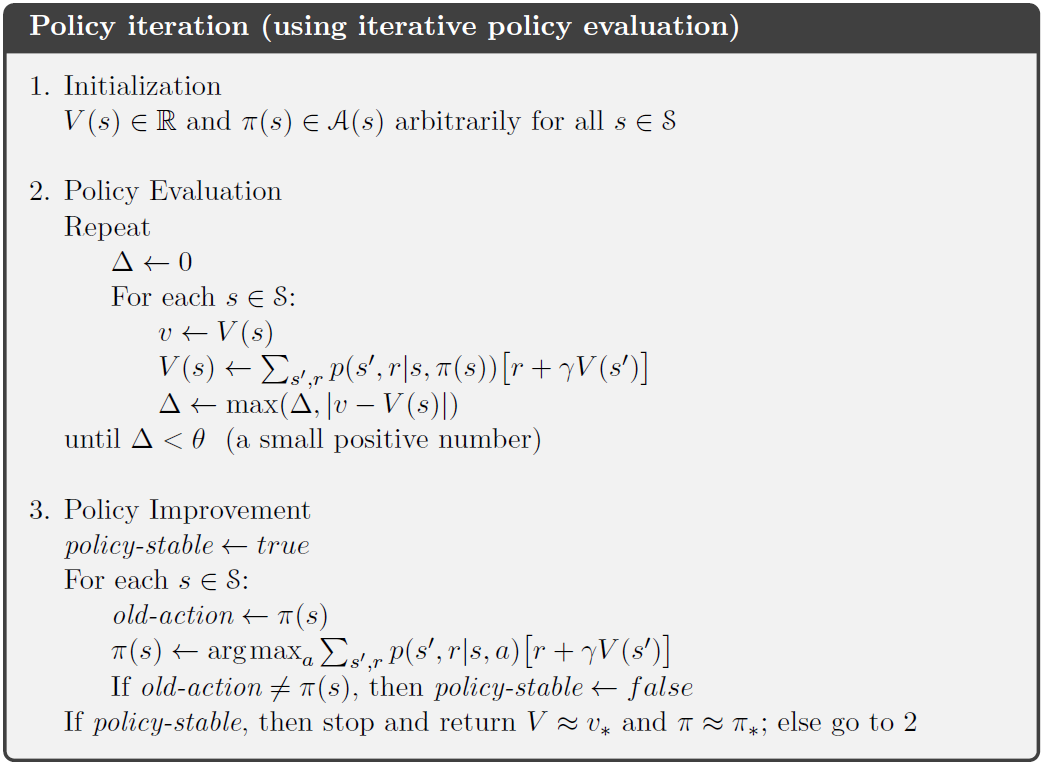4 Value Iteration
Policy iteration 的一个缺点是在每次迭代中都要进行 policy evaluation，这个过程是非常耗时的，事实上该过程是可以缩短的，并且不会影响收敛，其中一种重要的方法就是 Value Iteration，它可以写作是一个结合了 policy improvement 和删减的 policy evaluation 步骤的简单备份操作：

\begin{aligned} v_{k+1}(s) &\doteq \max_a {\Bbb E} [R_{t+1}+\gamma v_{k}(S_{t+1})| S_t=s,A_t=a] \\ &= \max_a \sum_{s',r}p(s',r|s,a)[r+\gamma v_{k}(s')] \end{aligned}
对任意的 $v_0$，序列 $\{v_k\}$ 最终都可以收敛到 $v_{\ast}$。

算法的伪代码如下：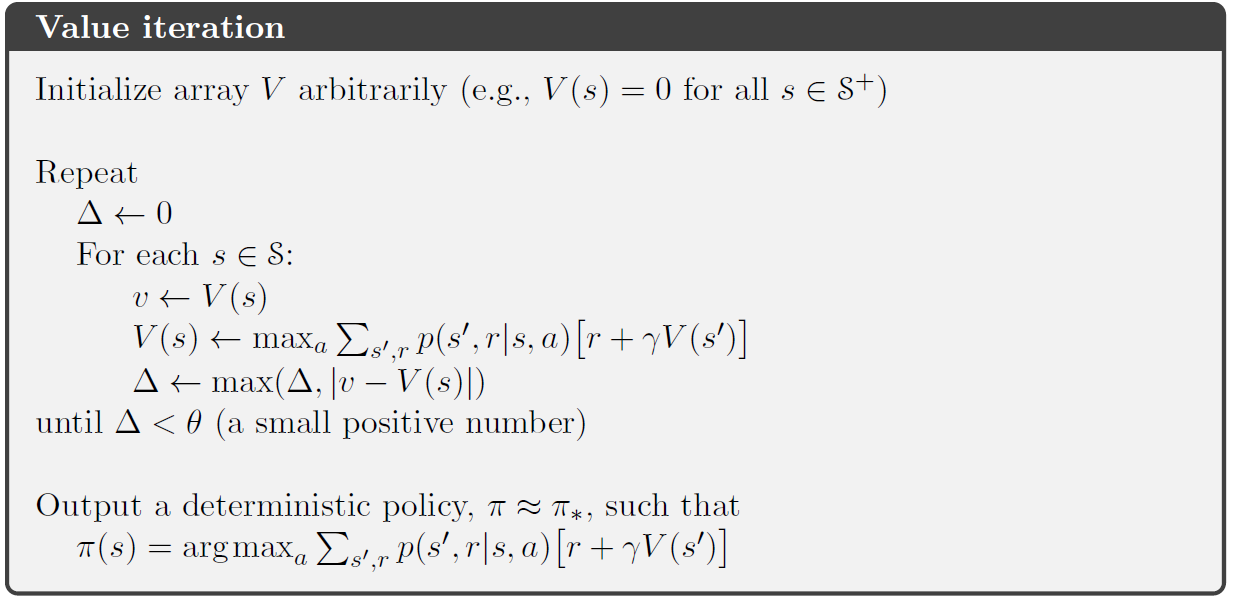所有的 truncated policy iteration 算法都可以视为是 sweep 序列，其中一些使用的是 policy evaluation backups，另一些使用的是 value iteration backups。
5 Asynchronous Dynamic Programming 异步的动态编程
DP方法的一个主要缺点就是在于它需要遍历 MDP 过程的整个状态集合，即需要对 state set 进行 sweep，当状态集合很大时，一次 sweep 就需要耗费巨大。
Asynchronous DP 方法替代了 iterative DP 算法，不是对状态集的系统地 sweep，这些方法会以任何顺序备份状态的 values，一些状态的 values 还有可能在其他状态第一次备份之前就备份了很多次。但为了正确收敛，异步算法必须继续备份所有的 values，异步 DP 在选择状态方面提供了很大的自由度。
6 Generalized Policy Iteration
广义的 policy iteration 可以用下图来表示：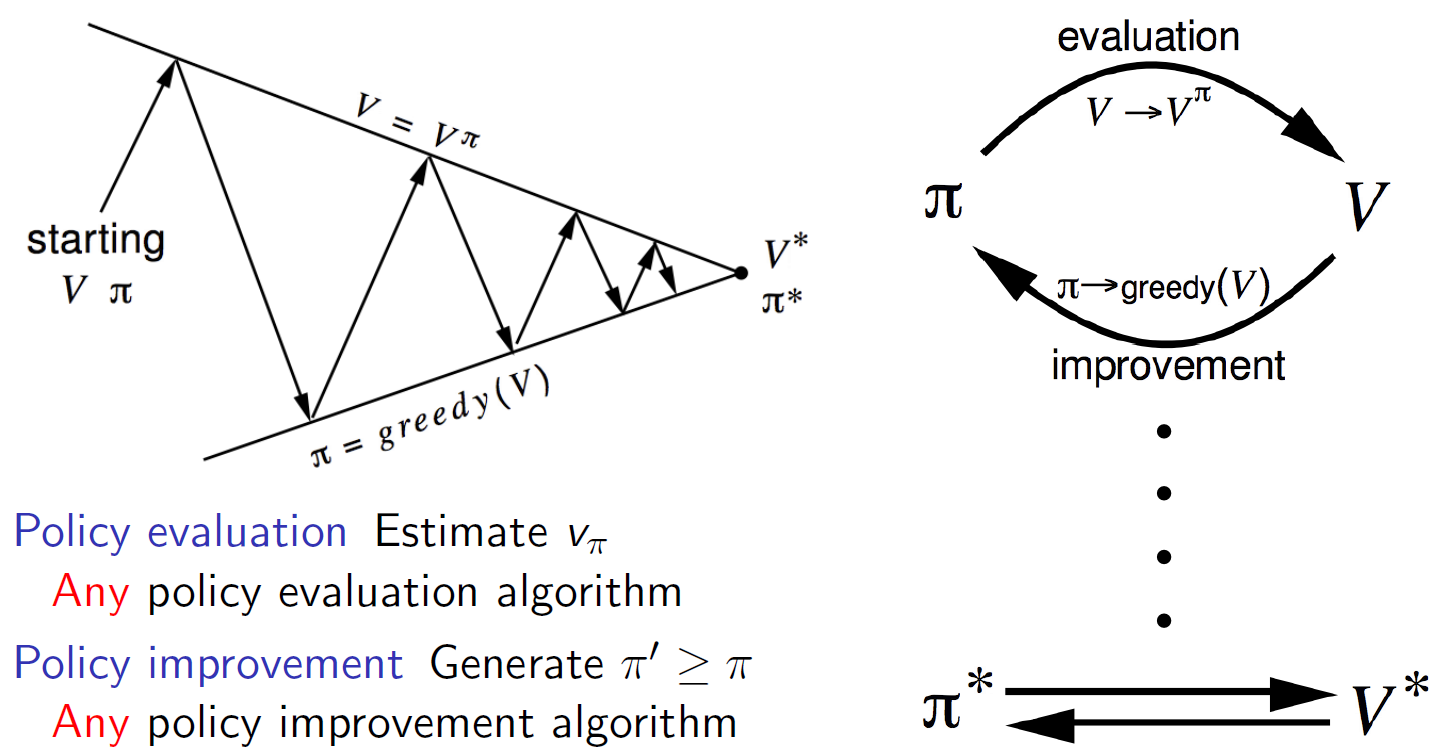Policy iteration 其实就是由两个连续的交互过程构成的：policy evaluation 和 policy improvement，在异步 DP 方法中，这两个过程是交错的。
这里用  Generalized Policy Iteration （GPI） 来代表这种一般化的思想，几乎所有的增强学习方法都可以描述为 GPI。在 GPI 中，policy evaluation 和 policy improvement 既是竞争的也是合作的。

参考文献
 Reinforcement Learning: An Introduction, Richard S. Sutton and Andrew G. Barto
 UCL Course on RL


展开全文算法
• ## Java动态编程初探

千次阅读 2019-06-19 11:28:33
作者简介 传恒，一个喜欢摄影和旅游的软件工程师，先后从事饿了么物流蜂鸟自配送和蜂鸟众包的开发，现在转战 Java，目前负责物流...简单的说就是在静态编程中，类型检查是在编译时完成的，而动态编程中类型检查是在...

作者简介
传恒，一个喜欢摄影和旅游的软件工程师，先后从事饿了么物流蜂鸟自配送和蜂鸟众包的开发，现在转战 Java，目前负责物流策略组分流相关业务的开发。

什么是动态编程
动态编程是相对于静态编程而言的，平时我们讨论比较多的静态编程语言例如Java，
与动态编程语言例如JavaScript相比，二者有什么明显的区别呢？
简单的说就是在静态编程中，类型检查是在编译时完成的，而动态编程中类型检查是在运行时完成的，
所谓动态编程就是绕过编译过程在运行时进行操作的技术。
动态编程使用场景

通过配置生成代码，减少重复编码，降低维护成本。
AOP的一种实现方式，方便实现性能监控和分析，日志，事务，权限校验等。
实现新语言的语义，例如Groovy使用ASM生成字节码。
单元测试中动态mock测试依赖。

在Java中有如下几种方式实现动态编程：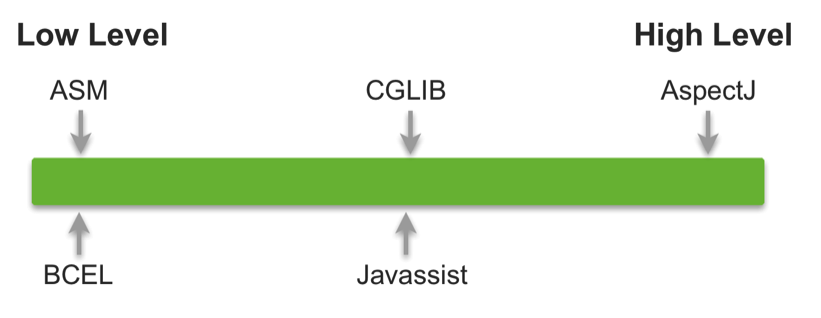反射
我们常用到的动态特性主要是反射，在运行时查找对象的属性和方法，修改作用域，通过方法名称调用方法等。在线的应用不建议频繁使用反射，因为反射的性能开销较大。
动态代理
在java的java.lang.reflect包下提供了一个Proxy类和一个InvocationHandler接口，通过这个类和这个接口可以生成JDK动态代理类和动态代理对象。
动态编译
动态编译是从Java 6开始支持的，主要是通过一个JavaCompiler接口来完成的。通过这种方式我们可以直接编译一个已经存在的java文件，也可以在内存中动态生成Java代码，动态编译执行。
调用Java Script引擎
Java 6加入了对Script(JSR223)的支持。这是一个脚本框架，提供了让脚本语言来访问Java内部的方法。你可以在运行的时候找到脚本引擎，然后调用这个引擎去执行脚本，这个脚本API允许你为脚本语言提供Java支持。
动态生成字节码
操作java字节码的工具有BECL/ASM/CGLIB/Javassist，其中有两个比较流行的，一个是ASM，一个是Javassist。
ASM直接操作字节码指令，执行效率高，要求使用者掌握Java类字节码文件格式及指令，对使用者的要求比较高。
Javassist提供了更高级的API，执行效率相对较差，但无需掌握字节码指令的知识，对使用者要求较低，所以接下来我们重点讲讲Javassist。
Javassist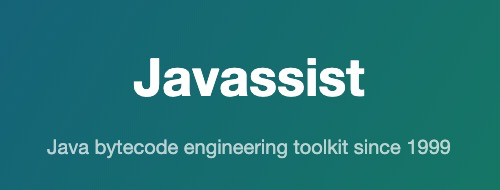Javassist是一个开源的分析、编辑和创建Java字节码的类库。
它是由东京工业大学的数学和计算机科学系的 Shigeru Chiba (千叶滋) 所创建的，目前已经加入到开放源代码JBoss应用服务器项目，JBoss通过使用Javassist对字节码进行操作，实现动态AOP框架。
Javassist(Java Programming Assistant) 使对Java字节码的操作变得简单，它使Java程序能够在运行时定义新类，并且可以在JVM加载时修改类文件。
与其它类似的字节码编辑器不同，它提供两个级别的API：源级别和字节码级别。
如果用户使用源级别API，他们可以在不知道Java字节码规范的情况下编辑类文件。整个API仅使用Java语言的词汇表进行设计，你甚至可以使用Java源代码的方式插入字节码。
另外，用户也可以使用字节码级别的API去直接编辑类文件。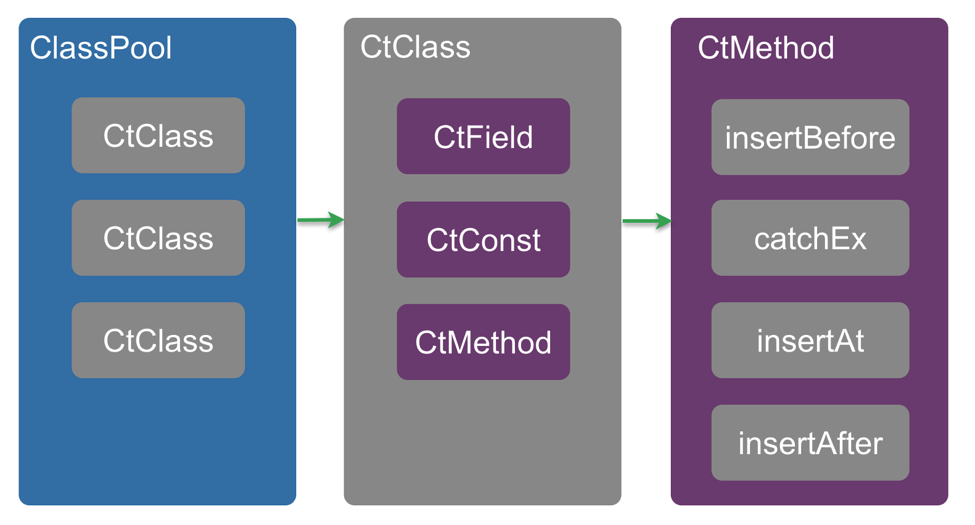// ClassPool 是 CtClass 对象的容器，存储着CtClass的Hash表。它按需读取类文件来构造CtClass对象，并且保存CtClass对象以便之后使用
ClassPool classPool = ClassPool.getDefault();
// CtClass 表示一个class文件，一个 GtClass(compile-time class)对象用来处理一个class文件，下面是从classpath中查找该类
CtClass ctClass = classPool.get("test.config.ConfigHandle");
// 通知编辑器去寻找对应的包
classPool.importPackage("org.mockito.Mockito");
classPool.importPackage("test.adapter.ext.IDowngrade");
classPool.importPackage("test.utils.property.IProperties");
// 使用removeField() removeMethod() 去删除对应的属性和方法
ctClass.removeField(ctClass.getDeclaredField("serviceHandle"));
ctClass.removeField(ctClass.getDeclaredField("switchHandle"));
ctClass.removeField(ctClass.getDeclaredField("configHandle"));
// CtMethod 和 CtConstructor 提供了 setBody() 方法去修改方法体
CtConstructor ctConstructor = ctClass.getDeclaredConstructors();
ctConstructor.setBody("{this.mySwitch = Mockito.mock(IDowngrade.class);\n" +
"            this.myConfig = Mockito.mock(IProperties.class);}");
// toClass() 请求当前线程的 ClassLoader 去加载 CtClass 所代表的类文件
ctClass.toClass();
//输出成二进制格式
//byte[] b = ctClass.toBytecode();
//输出class文件到目录中
//ctClass.writeFile("/tmp");


ClassPool是CtClass对象的容器，因为编译器在编译引用CtClass代表的Java类的源代码时，可能会引用CtClass对象，所以一旦一个CtClass被创建，它就被保存在ClassPool中。
如果事先知道要修改哪些类，修改类的最简单方法如下：

调用 ClassPool.get() 获取 CtClass 对象
修改对象
调用 CtClass 对象的 writeFile() 或者 toBytecode() 获得修改过的类文件。

如果需要定义一个新类，只需要
ClassPool pool = ClassPool.getDefault();
CtClass cc = pool.makeClass("HelloWorld");

冻结classes
如果一个 CtClass 对象通过 writeFile(), toClass(), toBytecode()被转换成一个类文件，该CtClass对象会被冻结起来，不允许再修改，因为一个类只能被JVM加载一次。
CtClasss cc = ...;
:
cc.writeFile();
cc.defrost();
cc.setSuperclass(...);    // 类已经被解冻

Class 搜索路径：
通过 ClassPool.getDefault() 获取的ClassPool默认使用JVM的类搜索路径。如果程序运行在JBoss或者Tomcat等Web服务器上，ClassPool可能无法找到用户自己定义的类，因为这种Web服务器使用多个类加载器作为系统类加载器。在这种情况下，ClassPool必须添加额外的类搜索路径。
pool.insertClassPath(new ClassClassPath(this.getClass())); // 当前的类使用的类路径，注册到类搜索路径
pool.insertClassPath("/usr/local/javalib"); // 添加目录 /usr/local/javalib 到类搜索路径
ClassPath cp = new URLClassPath("www.javassist.org", 80, "/java/", "org.javassist.");
pool.insertClassPath(cp); // 注册URL到搜索路径

在Java中，多个类加载器是可以共存的。每个类加载器创建了自己的命名空间，不同的类加载器可以加载具有相同类名的不同类文件，被加载的类也会被视为不同的类。此功能使我们能够在单个JVM上面运行多个应用程序，即使这些程序包含具有相同名称的类。
注意，JVM不允许动态重新加载类，一旦类加载器加载了一个类，就不能再在运行时重新加载该类的其它版本。因此，在JVM加载类之后，就不能再更改该类的定义。
但是，JPDA（Java平台调试器架构）提供有限的重新加载类的能力，如果相同的类文件由两个不同的类加载器加载，则JVM内会创建两个具有相同名称但是定义的不同的类。由于两个类不相同，所以一个类的实例不能被分配给另一个类的变量，两个类之间的转换操作也会失败并且抛出一个ClassCastException异常。
总结
Javassist比我们在本文中所讨论的功能要丰富得多，作为jboss的一个子项目，其主要的优点在于简单和快速，可以直接使用java编码的形式，而不需要了解虚拟机指令，就能动态改变类的结构，或者动态生成类。如果你不是很了解虚拟机指令，可以采用javassist。
参考文档：

https://www.javassist.org/tutorial/tutorial.html
https://en.wikipedia.org/wiki/Javassist

阅读博客还不过瘾？

欢迎大家扫二维码通过添加群助手，加入交流群，讨论和博客有关的技术问题，还可以和博主有更多互动博客转载、线下活动及合作等问题请邮件至 shadowfly_zyl@hotmail.com 进行沟通


展开全文java
• ## 秒懂Java动态编程（Javassist研究）

万次阅读 多人点赞 2018-07-30 13:44:27
动态编程是相对于静态编程而言的,平时我们讨论比较多的就是静态编程语言，例如Java，与动态编程语言，例如JavaScript。那二者有什么明显的区别呢？简单的说就是在静态编程中，类型检查是在编译时完成的，而动态编程...动态代理
• Dynamic Programming Language (动态语言或动态编程语言) 动态语言，准确地说，是指程序在运行时可以改变其结构：新的函数可以被引进，已有的函数可以被删除等在结构上的变化。比如众所周知的ECMAScript(JavaScript)...
• python是一种跨平台、开源、免费的高级动态编程语言，对。python具有简单、易学、速度快、免费、开源、可移植性、可扩展性、丰富的库等优点。python语言极其容易上手，它是一种代表简单主义思想的语言。python是一种...
• ## 动态编程语言

千次阅读 2009-12-31 17:30:00
动态编程语言（Dynamic Programming Language），在运行期间完成需要其它高级语言在编译器决定的一些行为，这些行为可以被任何高级语言在足够足够复杂的情况下实现，而动态变成语言可以直接使用工具来运用这种特性。...语言 closures 编译器 虚拟机
• 动态编程是相对于静态编程而言的,平时我们讨论比较多的就是静态编程语言，例如Java，与动态编程语言，例如JavaScript。那二者有什么明显的区别呢？简单的说就是在静态编程中，类型检查是在编译时完成的，而动态编程...Java
• Python是一门跨平台、开源、免费的解释型高级动态编程语言。Python支持命令式编程（How to do）、函数式编程（What to do），完全支持面向对象程序设计，拥有大量扩展库。胶水语言：可以把多种不同语言编写的程序...
• 动态编程是相对于静态编程而言的,平时我们讨论比较多的就是静态编程语言，例如Java，与动态编程语言，例如JavaScript。那二者有什么明显的区别呢？简单的说就是在静态编程中，类型检查是在编译时完成的，而动态编程...
• 定义  通常我们所说的动态语言、静态语言指 动态类型语言（Dynamically Typed Language）和 静态类型语言Statically...还有一个 Dynamic Programming Language （动态编程语言），静态编程语言。 动态类型语言：在运
• java算法：动态编程 分治法，简单的说就是把问题分成多个子问题，当子问题不独立时，情况就复杂了。 例1：斐波纳契数列 Java代码 static int f(int i){ if(i 1){ return 0; } if(i == 1){ ...
• ## 拓展动态编程的新领域

千次阅读 热门讨论 2010-08-18 15:13:00
拓展动态编程的新领域 ——C# 4动态编程新特性与DLR剖析语言 c#
• C# 4.0的主题就是动态编程(Dynamic Programming)。虽然C#仍然是一种静态语言，但是对象的意义开始变得越来越“动态”。它们的结构和行为无法通过静态类型来捕获，或者至少编译器在编译程序时无法得知对象的结构和...c# 编译器 string 语言
• 最近被这几个东西搞得晕头转向。而网上对这几个概念的阐述也是众说纷纭。 遇到这种问题时，我的做法是——回归英文。...动态编程语言：Dynamic programming language 静态编程语言： 参考文
• ## C++ 扩展动态编程

千次阅读 2006-09-04 01:11:00
C++规范不支持当前流行的动态编程（这是出于效率的性能考虑，使用的是早绑定的技术，在编译的时候内容就已经确定下来，运行时刻进行改变确实是一个痛苦的事情），但是这并不影响我们的程序设计。事实上，动态编程中...c++ 扩展 微软 class
• 　动态编程（Dynamic Programming, DP）这个词大家肯定都不陌生，在解决算法编程问题当中经常会用到，它的主要思想就是将一个复杂的问题分解成多个子问题，将子问题的解结合在一起就构成了原问题的解，它常常适合于...
• 1.动态编程  当分而治之算法划分的子问题不是相互独立时，直接的递归实现会因为子问题相互重叠导致惊人的多余计算和函数调用，甚至为指数递增  Example:用递归实现斐波那契序列      实现代码：  int ...C++数据结构
• 原文链接：http://tecdat.cn/?p=11105 在强化学习中，我们有兴趣确定一种最大化获取奖励的策略。假设环境是马尔可夫决策过程 （MDP）的理想模型 ，我们可以应用动态编程方法来解决强化学习问题。
• 概述　Javassist是一款字节码编辑工具，可以直接编辑和生成Java生成的...熟练使用这套工具，可以让Java编程更接近与动态语言编程。教程maven依赖pom.xml <groupId>org.javassist <artifactId>javassist</artifactId>javassist
• java可以在运行时，进行动态编译，也就是说，可以在代码中，由程序控制进行编译俩种动态编译方法： 1.执行cmd命令，调用javac进行编译 2.利用java提供的JavaComplier 3.可以利用类加载器还有java反射来执行编译好...java
• 本文使用一个简单实例讲述如何使用多态在Delphi中实现动态编程。 多态（Polymorphism）按字面的意思就是“多种形状”。引用Charlie Calverts对多态的描述——多态性是允许你将父对象设置成为和一个或更多的他的子...delphi shapes 语言
• 近几年来，在TIOBE公司每个月发布的编程语言排行榜中，C#... 1 动态编程语言的中兴 　动态编程语言并非什么新鲜事物，早在面向对象编程语言成为主流之前，人们就已经使用动态编程语言来开发了。即c# 微软 语言
• 动态编程指的是改变经典编程方式（源文件-->编译-->二进制文件---->运行方式）的行为. 1.使用Compiler Api 创建类文件，同时允许在程序运行中编译源文件。在动态使用java类时可以启用诊断信息监听器和编译...java javassist
•语言 c c++...